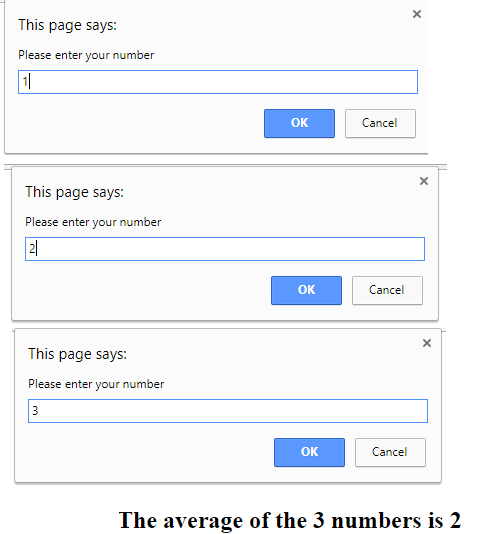### Loops

Counter-controlled loops used to repeat executing a JavaScript code a predefined number of times. A counter is used to control the number of times the code is being executed.

Here is an example of a JavaScript code illustrating loop calculating the average of 3 numbers entered by the user:

```	<!DOCTTYPE html>
<!--Example 1: Loop.html -->
<html>
<head>
<title> Average of 3 numbers </title>
<script >
<!--
var sum; //sum of numbers
var counter; //number of times the user enters numeric data and will not exceed the value 3 in this example
var number; //number entered by the user and read as a string
var converted_number; //entry converted to integer
var average;
sum = 0;
counter=1;
while (counter <=3)  //begining of the while loop
{
number = window.prompt("Please enter your number");
converted_number = parseInt(number); //convert into integer
sum = sum + converted_number; //add the number to sum
counter = counter + 1; // increment counter by 1
} //end of while
average = sum/3; //calculate the average
document.writeln('<h2>The average of the 3 numbers is ' + average + '<h2>');
// -->
</script>
</head>
<body></body>
</html>
```
Here is the output of this JavaScript program:For more details, please contact me here.
Date of last modification: March 26, 2019.